Raspberry Pi‎ > ‎

### Pi DiePi Die is a Rasberry Pi powered die. Give it a shake, and the die displays a random number from one to six. The number is displayed in a traditional die pattern using seven green LED's.What you need :7 x 5 mm green LED's.7 x 180 ohm resistor.1 x 10K resistor.1 x 1K resistor.1 x Tilt switch.Cardboard from a cereal box or similar.12 x Cables to connect to the piSolder and soldering iron.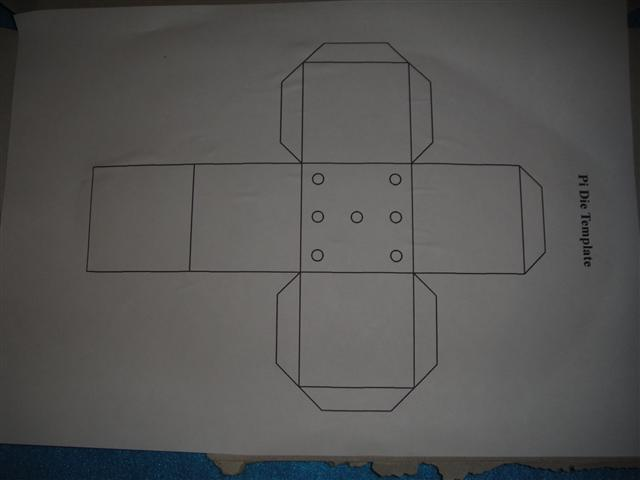Glue the template to the cereal box.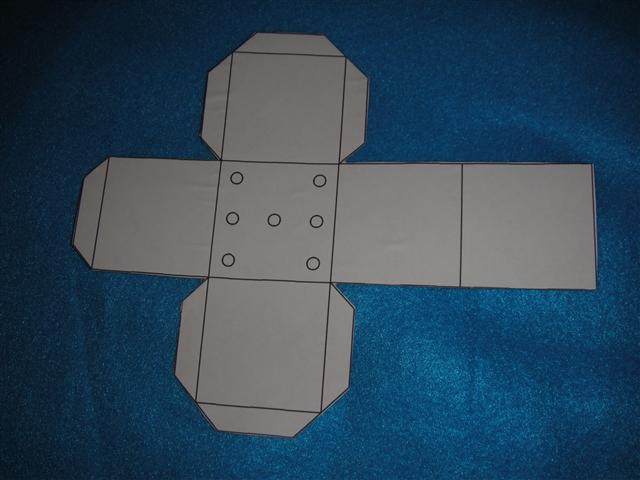Cut out around the outsidePierce holes for the LED's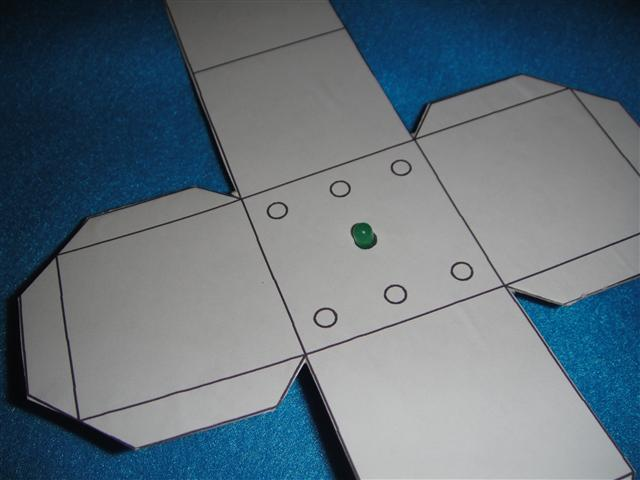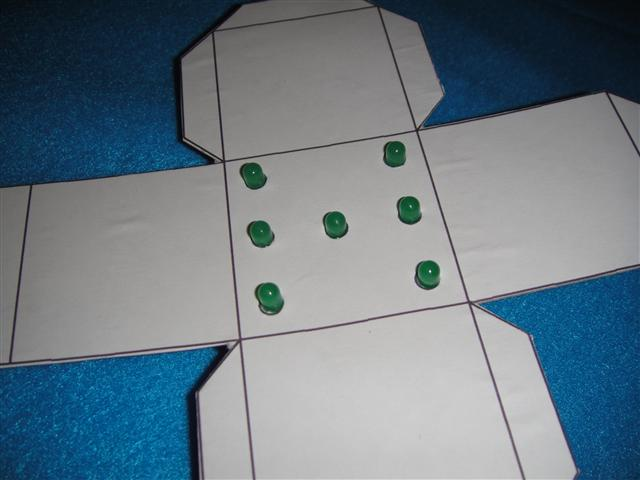Check that all of the LED's fit into the holesNext, we'll do the electronics. Here's the circuit diagram :Note the push button is actually the tilt switch. I didn't have a symbol for the tilt switch.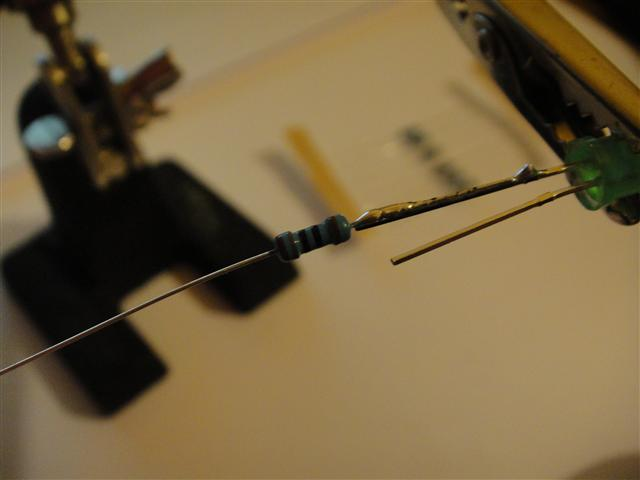Take the LED's and solder a 180 ohm resistor onto the longer lead of each one (anode)You should have seven LED's like this.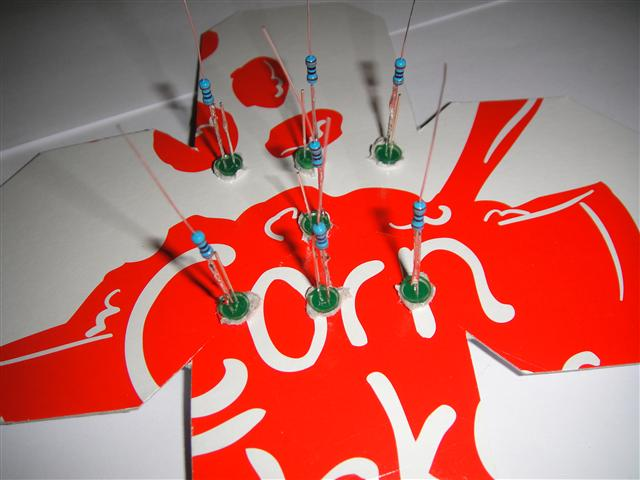Fit the LED's into the holes in the cardboard.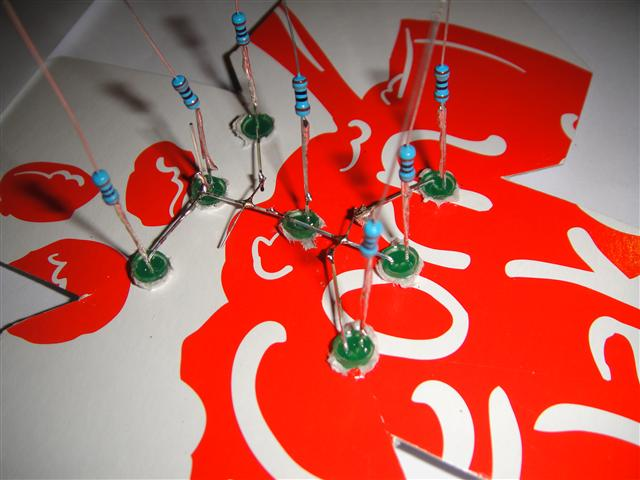Bend over the negative leads of the LED's and solder them all together. You'll probably find that some of them aren't quite long enough, so you'll need bridge the gap with some wire.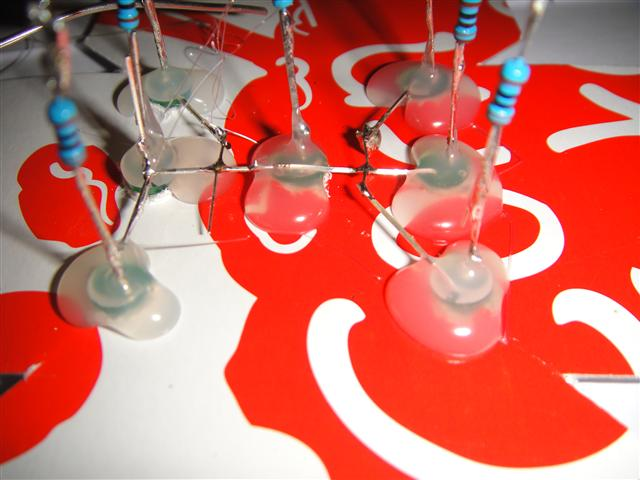Glue them into place using hot melt glue.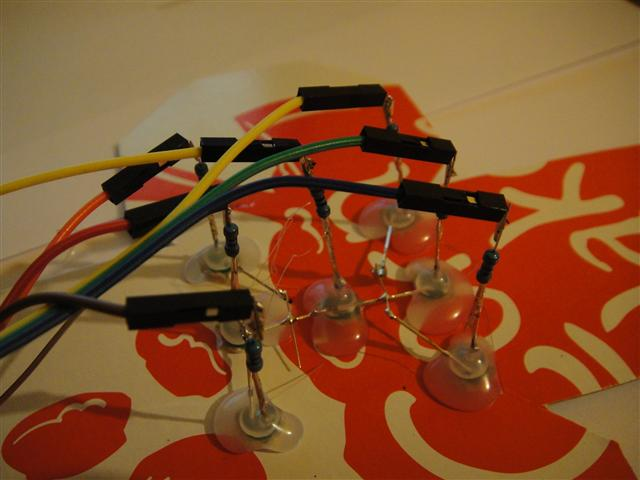Solder on cables to connect to the free ends of all of the resistors, as well as one for thenegative leads on the resistors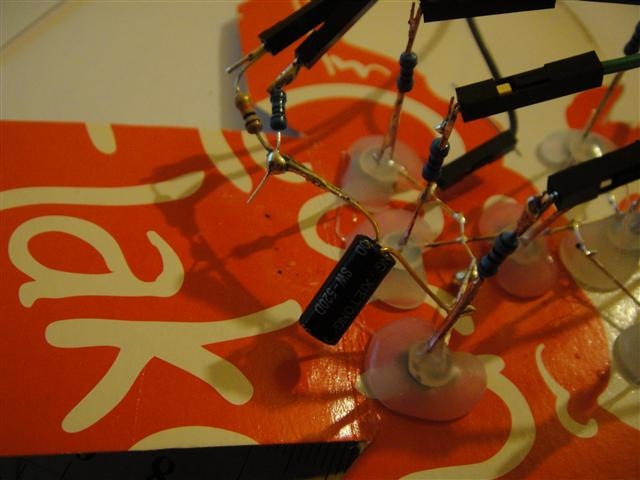Next, solder the switch and resistors in place. One lead of the switch is connected to ground (negative) the other lead is  connected to both the 10K resistor and the 1K resistor. The 10K resistor is to float the GPIO pin high. The 1K resistor stops the switch from shorting out the GPIO pin, killing the pi.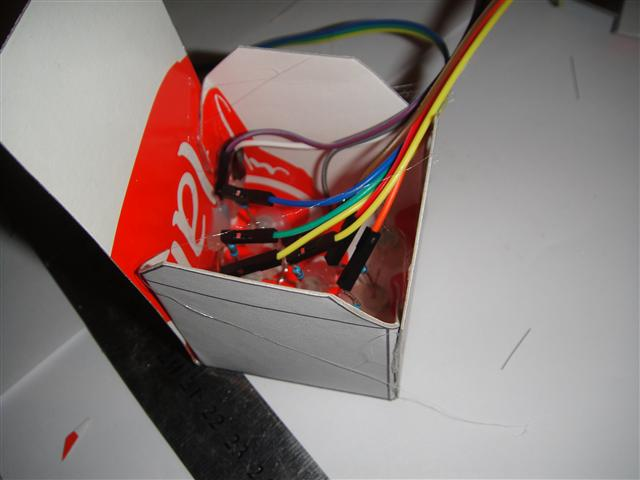Assemble the box.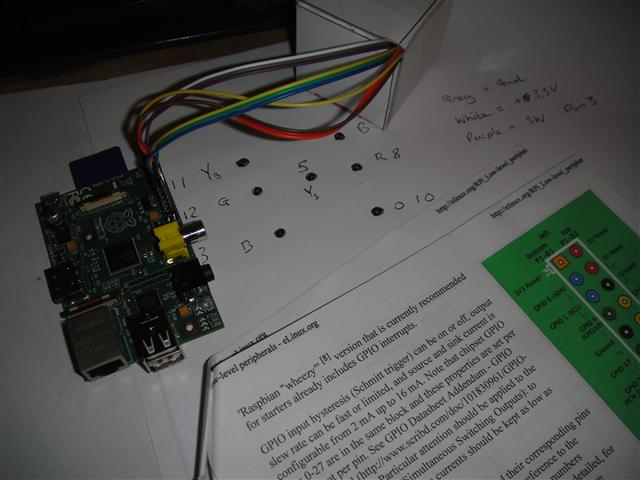Now to connect it all up. Make notes of which wires are connected to which LED's / switches and connect them to the appropriate GPIO pins.import RPi.GPIO as GPIOimport time as timeimport random as randomGPIO.setmode(GPIO.BOARD)GPIO.setwarnings(False)GPIO.setup (16, GPIO.IN)GPIO.setup (5, GPIO.OUT)GPIO.setup (7, GPIO.OUT)GPIO.setup (8, GPIO.OUT)GPIO.setup (10, GPIO.OUT)GPIO.setup (11, GPIO.OUT)GPIO.setup (12, GPIO.OUT)GPIO.setup (13, GPIO.OUT)GPIO.output (5, False)GPIO.output (7, False)GPIO.output (8, False)GPIO.output (10, False)GPIO.output (11, False)GPIO.output (12, False)GPIO.output (13, False)state1 = 0state2 = 0t = 0time2 = 0count = 0delay = 0.003number = 0number1 = 0while True:    state1 = GPIO.input(16)    time.sleep(0.06)    state2 = GPIO.input(16)    if state1 != state2:        time2 = 0        while time2 < 15:            GPIO.output (5, True)            GPIO.output (7, True)            GPIO.output (8, True)            GPIO.output (10, True)            GPIO.output (11, True)            GPIO.output (12, True)            GPIO.output (13, True)            time.sleep(delay)            GPIO.output (5, False)            GPIO.output (7, False)            GPIO.output (8, False)            GPIO.output (10, False)            GPIO.output (11, False)            GPIO.output (12, False)            GPIO.output (13, False)                time.sleep(delay)                state1 = GPIO.input(16)            time.sleep(0.05)            state2 = GPIO.input(16)            number1 = number            number = random.randrange(10000, 70000, 1)                if state1 != state2:                time2 = 0            else:                time2 +=1            if number < 20000:            GPIO.output (5, True)            time.sleep(1)        elif number < 30000:            GPIO.output (8, True)            GPIO.output (12, True)            time.sleep(1)        elif number < 40000:            GPIO.output (13, True)            GPIO.output (5, True)            GPIO.output (10, True)            time.sleep(1)        elif number < 50000:            GPIO.output (7, True)            GPIO.output (10, True)            GPIO.output (11, True)            GPIO.output (13, True)            time.sleep(1)        elif number < 60000:            GPIO.output (5, True)            GPIO.output (7, True)            GPIO.output (10, True)            GPIO.output (11, True)            GPIO.output (13, True)            time.sleep(1)        else:            GPIO.output (8, True)            GPIO.output (7, True)            GPIO.output (10, True)            GPIO.output (11, True)            GPIO.output (12, True)            GPIO.output (13, True)            time.sleep(1)GPIO.cleanup()Raspberry Pi is a trademark of the Raspberry Pi Foundation